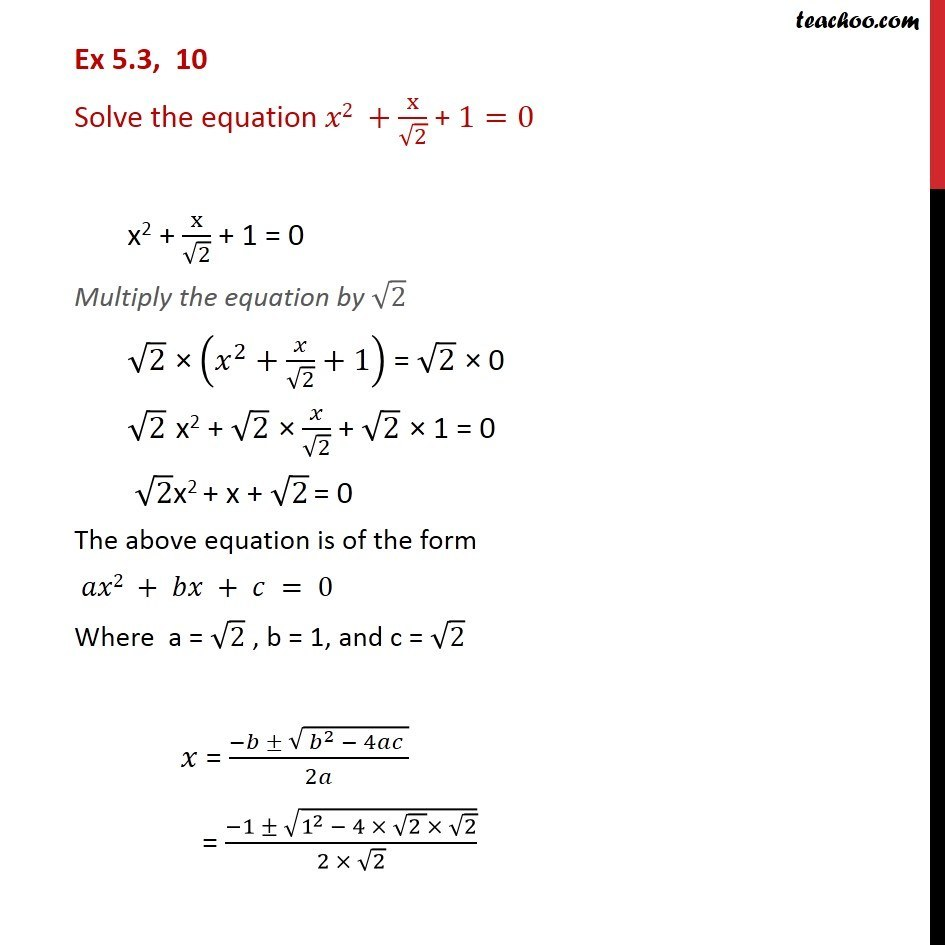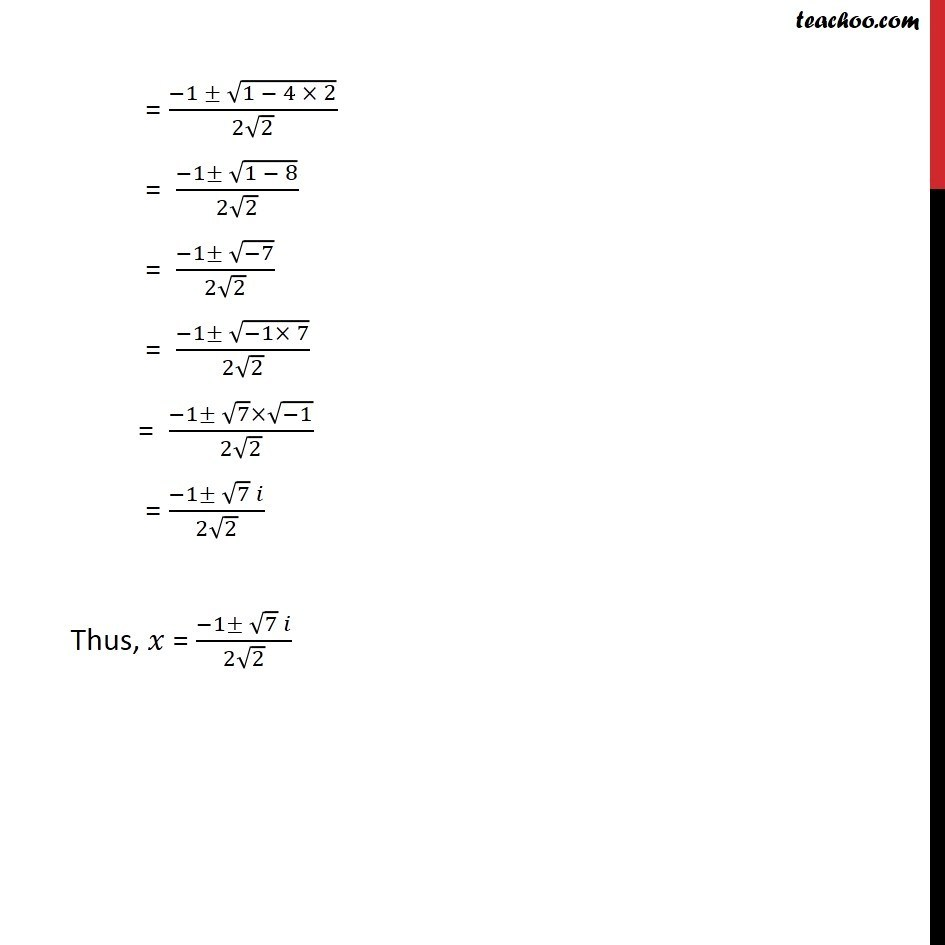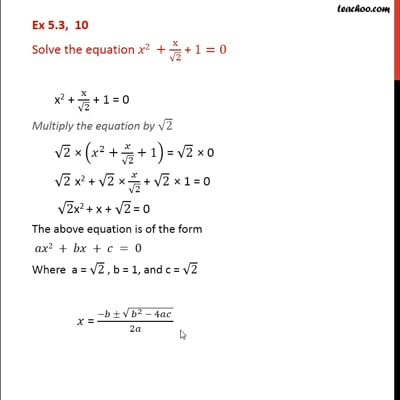Ex 5.3

Chapter 5 Class 11 Complex Numbers
Serial order wiseThis video is only available for Teachoo black users

Solve all your doubts with Teachoo Black (new monthly pack available now!)

### Transcript

Ex 5.3, 10 Solve the equation 2 + x/ 2 + 1=0 x2 + x/ 2 + 1 = 0 Multiply the equation by 2 2 ( ^2+ / 2+1) = 2 0 2 x2 + 2 / 2 + 2 1 = 0 2x2 + x + 2 = 0 The above equation is of the form 2 + + = 0 Where a = 2 , b = 1, and c = 2 = ( ( ^2 4 ))/2 = ( 1 (1^2 4 (2 ) 2) )/(2 2) = ( 1 (1 4 2))/(2 2) = ( 1 (1 8))/(2 2) = ( 1 ( 7))/(2 2) = ( 1 ( 1 7))/(2 2) = ( 1 7 ( 1))/(2 2) = ( 1 7 )/(2 2) Thus, = ( 1 7 )/(2 2)